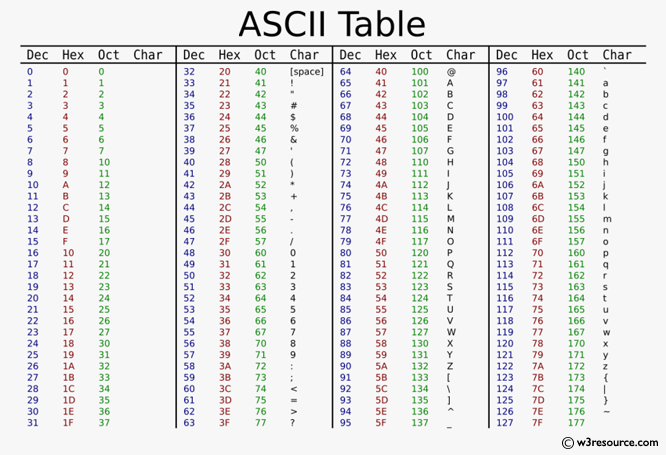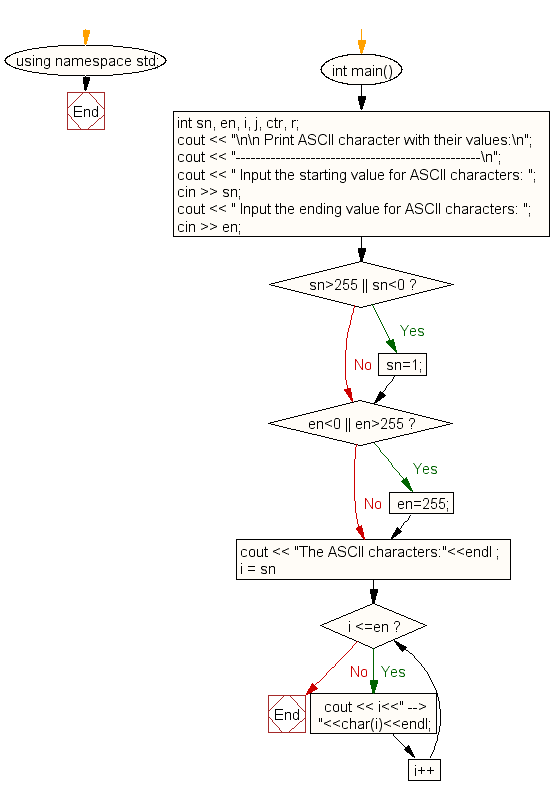﻿ C++ Exercises: Print all ASCII character with their values - w3resource

# C++ Exercises: Print all ASCII character with their values

## C++ For Loop: Exercise-61 with Solution

Write a C++ program that prints all ASCII characters with their values.

Pictorial Presentation:Sample Solution:-

C++ Code :

``````#include <iostream>
using namespace std;
int main()
{
int sn, en, i, j, ctr, r;
cout << "\n\n Print ASCII character with their values:\n";
cout << "-------------------------------------------------\n";
cout << " Input the starting value for ASCII characters: ";
cin >> sn;
cout << " Input the ending value for ASCII characters: ";
cin >> en;
if (sn>255 || sn<0)
sn=1;
if(en<0 || en>255)
en=255;
cout << "The ASCII characters:"<<endl ;
for (i = sn; i <=en; i++)
{
cout << i<<" --> "<<char(i)<<endl;
}
}
``````

Sample Output:

``` Print ASCII character with their values:
-------------------------------------------------
Input the starting value for ASCII characters: 65
Input the ending value for ASCII characters: 75
The ASCII characters:
65 --> A
66 --> B
67 --> C
68 --> D
69 --> E
70 --> F
71 --> G
72 --> H
73 --> I
74 --> J
75 --> K
```

Flowchart:C++ Code Editor: